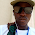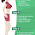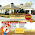Stella Dimoko Korkus.com: Lockdown Brain Teaser......

## Wednesday, April 08, 2020

### Lockdown Brain Teaser......

We may be locked down but our brains are still functioning.....right?

A house wife forgot her ATM pin. Which is four digit number, but luckily she remembered some hints on how to recall the pin. Here are some of the clues:

The first digit is half of the 2nd

The sum of 2nd and 3rd is 10

The 4th is equal to the 2nd plus 1

The sum of all the digit is 23.

*What is the atm pin ????*

#### 49 comments:

1.4829

I totally googled the answer.

1.Hehehehe. Funny you

2.3.I agree with whatever you say🤣🤣🤣

4.I agree with whatever you say🤣🤣🤣

5.I agree with whatever you say🤣🤣🤣

6.7.8.9.U are wicked 😂😂

2.I don't KNOW

3.The pin is ( 4 8 2 9 )

half of 8 is 4
8+2 = 10
8+1 = 9

4+8+2+9 = 23

😁😁😁😁😁

4.Brain sef don lockdown

1.2.3.Hehehehehehehehe

5.6.7.4829

I copied from Perxian
😂😂😂😂😂

1.🤣🤣🤣🤣🤣 and she copied from Google 🙆🙆🙆🙆

2.3.Copy copy student 😂😁😁😂

4.Hehehehehe

5.Brutally honest.
hahahaha
Perxian ... 1st olodo

Married chick ... 2nd olodo

6.8.9.4829.. I didn't google.. Was just maths.

10.4829

..... Essa Atelier’s client.

11.Let the numbers be A, B, C, D.
A= B/2
B+c =10
D=B+1
A+B+C+D=23
Solving a for B
(B/2 + 2B + 20-220-2B+2B+2)/2 = 23
3B + 22 = 46
3B = 46-22
3B = 24
B =24/3
B = 8
Therefore
A= 4
B =8
C= 2
D= 9
The numbers are 4,8,2,9

Jiji

1.Exactly how i solved mine..d love for maths tho!

12.Lol...its funny how she will forget 4 digits but remembers this complicated word algebraic puzzling Mathphysics

1.The thing tire me oh

2.Don't mind her

3.You dey mind her😂😂😂😂

13.14.4829

A=2B ,A=B/2 ...EQUATION 1
B+C=10
C=10-C.....2
D=B+1 ....3
A+B+C+D=23....4
SUBSTUTUTE FOR C AND D AND A IN EQUATION 4 WE GET
B/2 +B+(10-B)+(B+1)=23
THEREFORE 3B=24
B=8
FROM EQUATION 1
A=8/2=4
FROM EQUATION 3
B+C=10
C=10-8
C=2
FROM EQUATION 3
D=B+1
D= 8+1
9

PIN IS 4829
QED

15.Anything math, always gives me instant headache

1.That solution up there, give me 48 hrs, I won't understand. I hate maths.

16.17.The brain is on recess.😂😂

18.19.20.21.Abeg I no no, no scatter my brain this evening stella

22.The first digit there is 4,second is 8,3rd is 2 and 4th is 9.So the answer is 4829

23.The first digit there is 4,second is 8,3rd is 2 and 4th is 9.So the answer is 4829

24.8465
Four digits abcd
a/2 =b ....eq 1
b+c =10...eq2
d =b+1...eq3
a+b+c+d= 23...eq4

a =2b from 1
c= 10-b from2
Solve for b
2b+b+(10-b)+b+1=23
3b+b-b=23-10-1
3b=12
b=4
From 1..a=2b=2×4=8
From 2..b+c=10, c=10-4=6
From 3..d=b+1=4+1=5
From 4..a+b+c+d=23
8+4+6+5= 23

25.4829 I calculated within 50 mins

26.50secs actually

Disclaimer: Comments And Opinions On Any Part Of This Website Are Opinions Of The Blog Commenters Or Anonymous Persons And They Do Not Represent The Opinion Of StellaDimokoKorkus.com

Pictures and culled stories posted on this site are given credit and if a story is yours but credited to the wrong source,Please contact Stelladimokokorkus.com and corrections will be made..

If you have a complaint or a story,Please Contact StellaDimokoKorkus.com Via

Sdimokokorkus@gmail.com
Mobile Phone +4915210724141